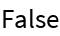#Function Repository Resource:

# InversionVectorQ

Check if a list is the inversion vector of a permutation written as a list

Contributed by: Wolfram Staff
 ResourceFunction["InversionVectorQ"][iv] checks if iv is the inversion vector of a permutation list.

## Details and Options

The inversion vector of a permutation of length n lists the number of times k is preceded by a number greater than k, where k runs from 1 to n.
The definition amounts to checking whether the entries of the vector are the digits of a number in base-factorial.

## Examples

### Basic Examples

This permutation has three numbers greater than 1 before 1, two numbers greater than 2 before 2 and so on: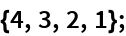Therefore this is its inversion vector: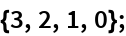Here is a check:

 In:=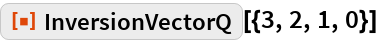Out=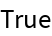The positive integer 23 gives {3,2,1,0} in the factorial base, so {3,2,1,0} is an inversion vector:

 In:=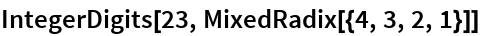Out=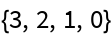Every inversion vector must end in 0 because the largest entry in a permutation list has nothing but smaller entries before it:

 In:=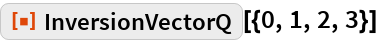Out=